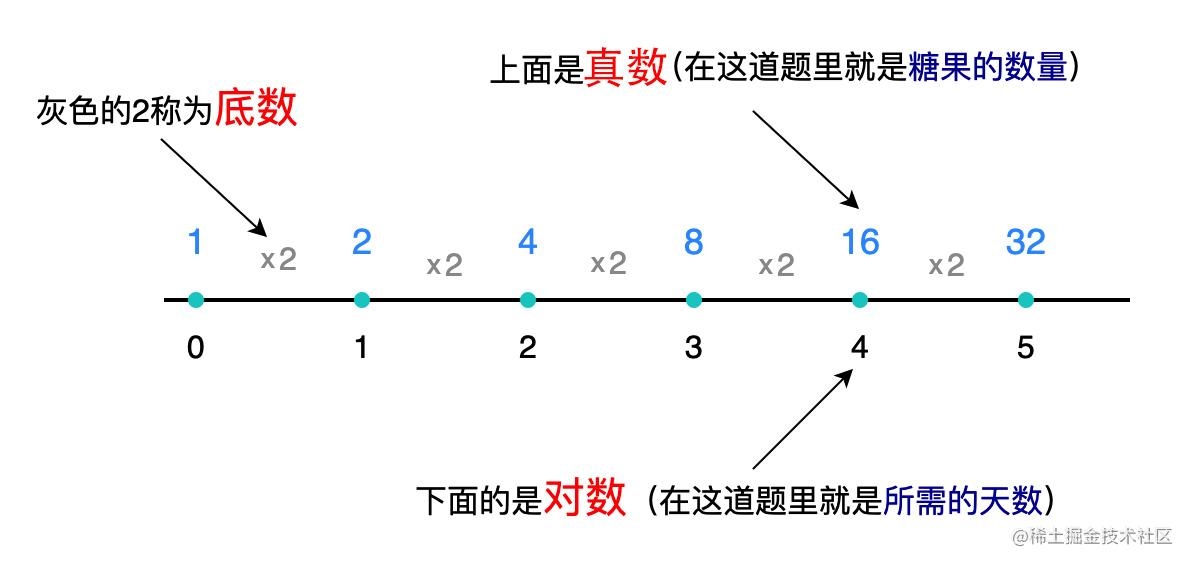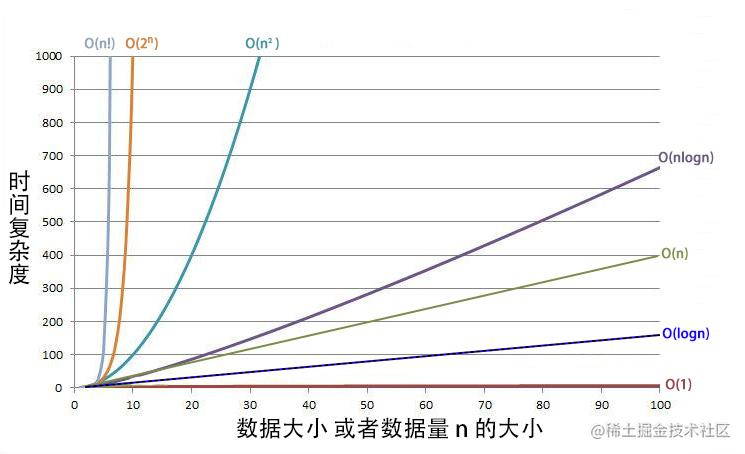# (算法入门)人人都能看懂的时间复杂度和空间复杂度

• 别人写的代码跑起来占内存 100M，耗时 100 毫秒
• 你写的代码跑起来占内存 500M，耗时 1000 毫秒，甚至更多

1. 衡量代码好坏有两个非常重要的标准就是：`运行时间``占用空间`，就是我们后面要说到的时间复杂度空间复杂度`也是学好算法的重要基石`
2. 这也是会算法和不会算法的攻城狮的区别、更是薪资的区别，因为待遇好的大厂面试基本都有算法

## 什么是时间复杂度

``````function foo1(){
console.log("我吃了一颗糖")
console.log("我又吃了一颗糖")
return "再吃一颗糖"
}

``````function foo2(n){
for( let i = 0; i < n; i++){
console.log("我吃了一颗糖")
}
return "一颗糖"
}

``````let = 0               ：执行 1 次
i < n                 : 执行 n+1 次
i++                   : 执行 n+1 次
console.log("执行了")  : 执行 n 次
return 1              : 执行 1 次

T(n) = O( f(n) )

``````n 是输入数据的大小或者输入数据的数量
T(n) 表示一段代码的总执行时间
f(n) 表示一段代码的总执行次数
O  表示代码的执行时间 T(n) 和 执行次数f(n) 成正比

• 第一个函数执行了3次，用复杂度表示就是 O(3)
• 第二个函数执行了3n + 4次，复杂度就是 O(3n+4)

• 如果只是常数直接估算为1，O(3) 的时间复杂度就是 `O(1)`，不是说只执行了1次，而是对常量级时间复杂度的一种表示法。一般情况下，只要算法里没有循环和递归，就算有上万行代码，时间复杂度也是O(1)
• O(3n+4) 里常数4对于总执行次数的几乎没有影响，直接忽略不计，系数 3 影响也不大，因为3n和n都是一个量级的，所以作为系数的常数3也估算为1或者可以理解为去掉系数，所以 O(3n+4) 的时间复杂度为 `O(n)`
• 如果是多项式，只需要保留n的最高次项`O( 666n³ + 666n² + n )`，这个复杂度里面的最高次项是n的3次方。因为随着n的增大，后面的项的增长远远不及n的最高次项大，所以低于这个次项的直接忽略不计，常数也忽略不计，简化后的`时间复杂度为 O(n³)`

## 常用时间复杂度

### O(1)

``````function foo(){
let n = 1
let b = n * 100
if(b === 100){
console.log("开始吃糖")
}
console.log("我吃了1颗糖")
console.log("我吃了2颗糖")
......
console.log("我吃了10000颗糖")
}

### O(n)

``````function foo1(n){
for( let i = 0; i < n; i++){
console.log("我吃了一颗糖")
}
}
function foo2(n){
while( --n > 0){
console.log("我吃了一颗糖")
}
}
function foo3(n){
console.log("我吃了一颗糖")
--n > 0 && foo3(n)
}

### O(n²)

``````function foo1(n){
for( let i = 0; i < n; i++){
for( let j = 0; j < n; j++){
console.log("我吃了一颗糖")
}
}
}

``````function foo2(n){
for( let k = 0; k < n; k++){
console.log("我吃了一颗糖")
}
for( let i = 0; i < n; i++){
for( let j = 0; j < n; j++){
console.log("我吃了一颗糖")
}
}
}

//或者下面这样，以运行时间最长的，作为时间复杂度的依据，所以下面的时间复杂度就是 O(n²)
function foo3(n){
if( n > 100){
for( let k = 0; k < n; k++){
console.log("我吃了一颗糖")
}
}else{
for( let i = 0; i < n; i++){
for( let j = 0; j < n; j++){
console.log("我吃了一颗糖")
}
}
}
}

### O(logn)``````function foo1(n){
let day = 0
while(n > 1){
n = n/2
day++
}
return day
}
console.log( foo1(16) ) // 4

``````function foo2(n){
for(let i = 0; i < n; i *= 2){
console.log("一天")
}
}
foo2( 16 )• `真数`：就是真数，这道题里就是16
• `底数`：就是值变化的规律，比如每次循环都是i*=2，这个乘以2就是规律。比如1,2,3,4,5...这样的值的话，底就是1，每个数变化的规律是+1嘛
• `对数`：在这道题里可以理解成x2乘了多少次，这个次数

ab = n  读作以a为底,b的对数=n，在这道题里我们知道a和n的值，也就是  2b = 16 然后求 b

logan = b    在这道题里就是   log216 = ?  答案就是 4

emmmmm.....

`O(1)`常数复杂度
`O(logn)`对数复杂度
`O(n)`线性时间复杂度
`O(nlogn)`线性对数复杂度
`O(n²)`平方
`O(n³)`立方
`O(2``n``)`指数，一点数据量就卡的不行
`O(n!)`阶乘，就更慢了## 什么是空间复杂度

### O(1)

``````function foo(){
let n = 1
let b = n * 100
if(b === 100){
console.log("开始吃糖")
}
console.log("我吃了1颗糖")
console.log("我吃了2颗糖")
......
console.log("我吃了10000颗糖")
}

### O(n)

``````function foo(n){
let arr = []
for( let i = 1; i < n; i++ ) {
arr[i] = i
}
}

### O(n²)

O(n²) 这种空间复杂度一般出现在比如二维数组，或是矩阵的情况下

``````let arr = [
[1,2,3,4,5],
[1,2,3,4,5],
[1,2,3,4,5]
]